• 既然是随机变量，当然就会有均值和标准差。 如果资产数量不是只有一个的话（一个的话，做什么资产配置），也就是存在有多个随机变量，随机变量之间当然就会有协方差。 资产配置的目的就是，找到一种较好的资产配置...
MPT
MPT, modern portfolio theory。现在资产配置理论。
理论很简单。
假设每个资产的收益率是一个随机变量$x_i$。既然是随机变量，当然就会有均值和标准差。
如果资产数量不是只有一个的话（一个的话，做什么资产配置），也就是存在有多个随机变量，随机变量之间当然就会有协方差。
资产配置的目的就是，找到一种较好的资产配置组合，使得达到预期的收益率的情况下，风险最小。
这句话其实就已经告诉了我们这个模型该如何建立。
建模
$E(r_w) = \sum_{i=1}^{n}{w_ir_i}$
$Var(r_w) = \sum_{i=1}^{n}{\sum_{j=1}^{n}{w_iw_jCov(r_i,r_j)}}$
我们根据上面的加粗文字就可以知道模型应该为：
$\min_{w}{Var(r_w)}\\ \mathrm{ s.t. } \qquad E(r_w) = \mu \\ \sum_{i=1}^{n}{w_i} = 1$

上面的模型中的约束如果不加上w应该大于等于0的话，就表示可以卖空，做负的配置。但是做负的配置的时候一般也是有保证金什么的。模型会复杂点。
最简单的情况是，考虑到w大于等于0

优化
优化的话，这里使用粒子群优化的方式。
其实一般最常用的是拉格朗日乘子法。
（拉格朗日乘子法是优化的最基础的算法啦，大家直接查就好了。这里我直接截的附件中的图）

最后用的是下面这个来解出拉格朗日算子。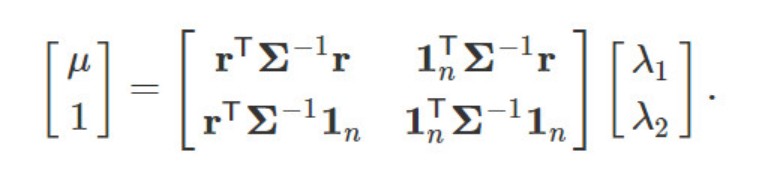代入到使得梯度为0的方程解出来的方程中。粒子群优化
粒子群的思路很简单，就是给一个初始化的向量。然后，每个粒子记住自己的历史最优解和全局的最优解。每次的迭代往这两个方向上加权的偏移就好了。
项目代码
假设有n个产品。cor是它们之间的协方差矩阵。然后，很明显在这个社会中风险越高（方差越大），那么这个产品的收益率越高。
给一个预期的期望收益。由于数据都是随机生成的。这里我们就直接取用在最大和0之间的alpha比例的数值（资产配置不可能高过最高均值收益）

给定$\alpha$,表示在最大和0之间的百分比。alpha取1表示最大值。0表示0。

n = 10
alpha = 0.8
cor = np.random.random((n, n))
mu = np.diag(cor)
EV = alpha * max(mu)


添加下面这两个函数，是为了保证生成的分配方式会使得期望收益达到目标设置的值。

def randOne(n):
a = np.random.random(n)
return a / np.sum(a)

def ReCheck(newData, n=10, oriData=None):
for i in range(len(newData)):
if oriData is None:
while np.matmul(newData[i], mu.T) < EV:
newData[i] = randOne(n)
elif np.matmul(newData[i], mu.T) < EV:
newData[i] = oriData[i]
return newData


m表示有m个粒子来做探索。对其做初始化
MTime 表示最大迭代周期

m = 30
por = np.random.random((m, n))
por = por / np.sum(por, axis=1)[:, np.newaxis]  # 归一

por = ReCheck(por)
w, c1, c2 = 0.6, 2, 2
v = np.random.random((m, n))
MTime = 500


计算数值（Objective function）

def CalVal(por):
tmp = 0
for i in range(len(por)):
for j in range(len(por)):
tmp += por[i] * por[j] * cor[i][j]
return tmp


粒子群迭代(解释思路)

一开始先算出每种分配所对应的总风险
然后最初的话，局部最优的结果当然就是初始化的结果。
目前已知的全局最优解，一样也就是这些局部最优解的最优解
迭代更新速度向量v。这个向量一开始也是随机初始化的。
有一个惯性因子w，即表示保持原来的方式飞行。
c1是局部最优因子;c2是全局最优因子
更新好速度向量后。再和原来相加。最后判断对应的数值有没有超过设置的预期均值。

t = 0
while t < MTime:
t += 1
val = np.zeros(m)
for i, p in enumerate(por):
val[i] = CalVal(p)

if t == 1:
local_Min_por = por.copy()
local_Min_val = val.copy()
else:
for i in range(m):
if val[i] < local_Min_val[i]:
local_Min_val[i] = val[i]
local_Min_por[i] = por[i]
global_Min_index = np.argmin(local_Min_val)
global_Min_por = local_Min_por[global_Min_index]
global_Min_val = local_Min_val[global_Min_index]

for i in range(m):
v[i] = w * v[i] + c1 * np.random.rand() * local_Min_por[i] + c2 * np.random.rand() * global_Min_por
new_por = por + v[i]
new_por = new_por / np.sum(new_por, axis=1)[:, np.newaxis]
por = ReCheck(new_por, oriData=por)

效果检验（和蒙特卡洛方法对比）
蒙特卡洛采用完全随机的方式。这里同样的设置总的次数相等。然后再通过不满足对应的期望收益标准的方案就去掉的方式。
random_por = np.random.random((m * MTime, n))
random_por = random_por / np.sum(random_por, axis=1)[:, np.newaxis]  # 归一random_


check and abandon

def ReCheck_abandon(newData):
ansData = newData.copy()
i, j = 0, 0
while i < len(newData):
if np.matmul(newData[i], mu.T) >= EV:
ansData[j] = newData[i]
j += 1
i += 1
return ansData[:j]
random_por = ReCheck_abandon(random_por)

val = np.zeros(m * MTime)[:len(random_por)]
for i, p in enumerate(random_por):
val[i] = CalVal(p)


画图

plt.plot(val)
plt.plot([global_Min_val for i in range(len(val))])


发现其实存在有不少数结果都比粒子群的方式要好。毕竟粒子群在这里关于粒子移动的速度的设定其实在这种求和受限制的空间的搜索效果并不太好。当然可以适当改进下。（欢迎大家提供改进方案，一起讨论~）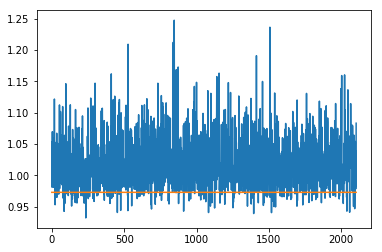展开全文马科维茨
• PortOpt [Portfolio Optimizer]是一个C ++程序（具有Python绑定），实现了Markowitz（1952）平均方差模型，该模型具有针对风险的代理线性无差异曲线，以便找到处于风险中的最优资产组合。 您必须提供PortOpt（在文本...开源软件
• 用的是米筐的研究模块，从结果来看，均值方差模型对参数的敏感性很高，很多参数都不如随机权重，很难应用到实战。 import pandas as pd import numpy as np from scipy import linalg import matplotlib.pyplot as ...
用的是米筐的研究模块，从结果来看，均值方差模型对参数的敏感性很高，很多参数都不如随机权重，很难应用到实战。
import pandas as pd
import numpy as np
from scipy import linalg
import matplotlib.pyplot as plt

stockslist = ['000001.XSHE','000002.XSHE','600004.XSHG','600033.XSHG','000651.XSHE']
data = get_price_change_rate(stockslist,start_date = '20100101',end_date = '20200420')
data.to_csv('MeanVar.csv')
print(data.head(20))
train_set = data[data.index<'20171231']
#print(train_set.tail(20))
test_set = data[data.index>'20180101']
#print(test_set.head(20))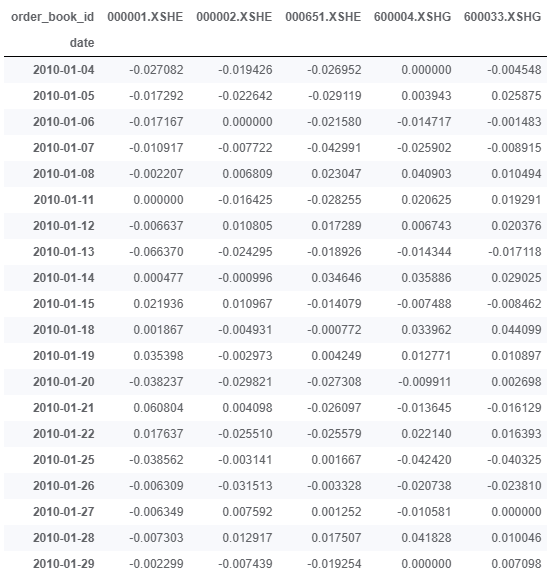#看一下5个股票的历史表现
cumreturn = (1 + data).cumprod()
cumreturn.plot()
plt.title('Cumulative Return of Stocks')
plt.show()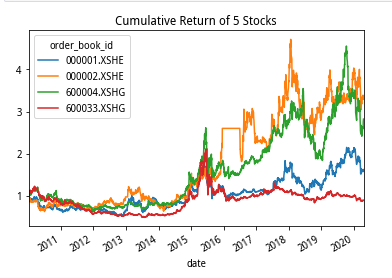#相关性分析
data.corr()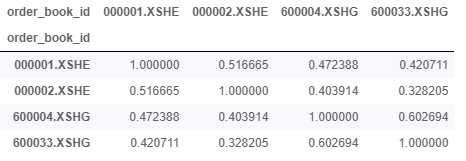#核心模块，实现均值方差模型的计算
class MeanVariance:
#传入收益率数据
def __init__(self,returns):
self.returns = returns
#定义最小化方差函数，即求解二次规划
def minVar(self,goalRet):
covs = np.array(self.returns.cov())
means = np.array(self.returns.mean())
L1 = np.append(np.append(covs.swapaxes(0,1),[means],0),
[np.ones(len(means))],0).swapaxes(0,1)
L2 = list(np.ones(len(means)))
L2.extend([0,0])
L3 = list(means)
L3.extend([0,0])
L4 = np.array([L2,L3])
L = np.append(L1,L4,0)
results = linalg.solve(L,np.append(np.zeros(len(means)),[1,goalRet],0))
return np.array([list(self.returns.columns),results[:-2]])
#定义绘制最小方差前缘曲线函数
def frontierCurve(self):
goals = [x/500000 for x in range(-100,4000)]
variances = list(map(lambda x: self.calVar(self.minVar(x)[1,:].astype(np.float)),goals))
plt.plot(variances,goals)
#定义各资产比例，计算收益率均值
def meanRet(self,fracs):
meanRisky = ffn.to_returns(self.returns).mean()
#assert (len(meanRisky == len(fracs),'Length of fractions must be equal to number of assets')
return np.sum(np.multiply(meanRisky,np.array(fracs)))
#定义各资产比例，计算收益率方差
def calVar(self,fracs):
return np.dot(np.dot(fracs,self.returns.cov()),fracs)

#计算有效前缘
minVar = MeanVariance(data)
minVar.frontierCurve()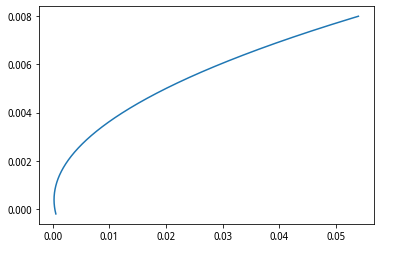#计算训练集权重
varMinimizer = MeanVariance(train_set)
goal_return = 0.003
portfolio_weight = varMinimizer.minVar(goal_return)
portfolio_weight

#计算测试集收益率
test_return = np.dot(test_set,np.array([portfolio_weight[1,:].astype(np.float)]).swapaxes(0,1))
test_return = pd.DataFrame(test_return,index = test_set.index)
test_cum_return = (1+test_return).cumprod()

#计算随机权重组合
sim_weight = np.random.uniform(0,1,(100,len(stockslist)))
sim_weight = np.apply_along_axis(lambda x: x/sum(x),1,sim_weight)
sim_return = np.dot(test_set,sim_weight.swapaxes(0,1))
sim_return = pd.DataFrame(sim_return,index = test_cum_return.index)
sim_cum_return = (1+sim_return).cumprod()

plt.plot(sim_cum_return.index,sim_cum_return,color = 'green')
plt.plot(test_cum_return.index,test_cum_return,label = 'MeanVar')
plt.legend()
plt.title('MeanVar & Random')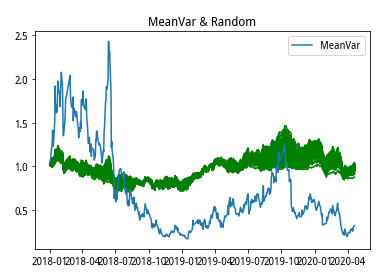展开全文• 常用的数据标准化方法有最大最小归一化、均值方差标准化、小数定标法、定量特征二值化等。其中，均值方差标准化是一种将数据转化为标准正态分布的标准化方法。在回归模型中，服从正态分布的自变量和因变量往往对应着...
一、原理介绍
常用的数据标准化方法有最大最小归一化、均值方差标准化、小数定标法、定量特征二值化等。其中，均值方差标准化是一种将数据转化为标准正态分布的标准化方法。在回归模型中，服从正态分布的自变量和因变量往往对应着较好的回归预测效果。均值方差标准化的计算公式为： $x'=\frac{x-\mu }{\sigma }$
公式中，x表示单个数据的取值，$\mu$表示对应列的均值，$\sigma$表示对应列的标准差
二、代码实现
#加载模块
from sklearn.preprocessing import StandardScaler
import warnings
warnings.filterwarnings("ignore") #过滤掉警告的意思
from pyforest import *
import pandas as pd
import numpy as np

#读入数据
data=pd.read_csv("F:/data/data.csv",encoding='gbk') #bgk表示中文编码
#查看数据前五行
data.head()


(1)资产负债率
(2)剔除预收款项后的资产负债率
(3)长期资本负债率
(4)长期资产适合率
(5)权益乘数

0
0.655799
0.606708
0.613865
0.494960
0.791971

1
0.752061
0.705498
0.841639
1.000000
0.932990

2
0.957391
0.941543
0.000000
0.493465
0.988630

3
0.807046
0.780709
0.984696
0.530637
0.958086

4
0.805235
0.796071
0.861480
0.541299
0.957462

#标准化，返回值为标准化后的数据
Standard_data=StandardScaler().fit_transform(data)
Standard_data


array([[-8.07868584e-01, -4.46781853e-01, -8.65101276e-02,
-2.18550266e-01, -4.97556164e-01],
[ 5.83511306e-02,  1.83702105e-01,  6.61977686e-01,
7.51432528e+00,  3.06672601e-01],
[ 1.90602996e+00,  1.69014811e+00, -2.10373034e+00,
-2.41440984e-01,  6.23989972e-01],
…,
[-1.14289225e-01, -1.81738238e-01, -4.01819153e-01,
-1.78750448e-04,  2.31292440e-01],
[-7.54465400e-01, -7.37349652e-01,  3.84582589e-01,
-2.41868662e-01, -3.87772198e-01],
[ 1.28886801e+00,  1.12495300e+00, -2.10373034e+00,
-2.48967045e-01,  5.66083685e-01]])

#由于标准化后的数据是array格式，故将其转化为数据框
Standard_data = pd.DataFrame(Standard_data) #转为dataframe

#将数据写成csv文件，方便后续的建模
Standard_data.to_csv("F:/data/Standard_data.csv",index=False)

下面验证标准化后的数据是否服从正态分布，这里以第一列数据为例
#为标准化后的数据加上列名
Standard_data.columns=data.columns
Standard_data.head()


(1)资产负债率
(2)剔除预收款项后的资产负债率
(3)长期资本负债率
(4)长期资产适合率
(5)权益乘数

0
-0.807869
-0.446782
-0.086510
-0.218550
-0.497556

1
0.058351
0.183702
0.661978
7.514325
0.306673

2
1.906030
1.690148
-2.103730
-0.241441
0.623990

3
0.553135
0.663697
1.132078
0.327724
0.449795

4
0.536841
0.761739
0.727179
0.490969
0.446237

#绘制概率密度图，用于展示数据分布
plt.rcParams['font.sans-serif']=['SimHei'] #图片显示中文
plt.rcParams['axes.unicode_minus'] =False #减号unicode编码
sns.distplot(Standard_data['(1)资产负债率'])
plt.show()从概率密度图中可以看出，标准化后的数据对称分布在0的两侧，接近于正态分布。


展开全文机器学习 数据分析 数据挖掘 csv
• 作者：chen_h 微信号 &amp;amp;amp; QQ：862251340 微信公众号：coderpai ...第一篇：计算股票回报率，均值方差 ...我们从收益率，均值方差开始学习，你可能认为计算这些值非常简单，但是...
作者：chen_h
微信号 & QQ：862251340
微信公众号：coderpai

第一篇：计算股票回报率，均值和方差
第二篇：简单线性回归
第三篇：多元线性回归和残差分析
第四篇：现代投资组合理论
第五篇：市场风险
第六篇：Fama-French 多因子模型

介绍
在本文中，我们将介绍有关量化金融的一些基本概念。我们从收益率，均值和方差开始学习，你可能认为计算这些值非常简单，但是，这些值是能用很多不同的方法来进行计算的。根据具体情况选择合适的计算方法是非常重要的。
回报率
单期回报率
单期（一个时期）的收益率计算方法如下：
$r=\frac{p_{t}}{p_{0}}-1=\frac{p_{t}-p_{0}}{p_{0}}$
其中，r 是收益率，pt 是时间 t 时的资产价格，p0 是初始时间的资产价格。我们利用 Python 程序来实现这个过程：
import numpy as np
rate_return = 102.0/100 - 1
print(rate_return)

假设我们买了 100 美元的股票，半年后它涨到了 102 美元。一年后，价格又涨到了 104 美元。那么我们应该如何计算它的总回报呢？首先，我们可以把它看做是一个时期的投资回报，即股票直接从 100 美元涨到了 104 美元，那么我们的回报率可以这样计算：
$r = \frac{104}{100} - 1 = 0.04$
或者，我们也可以把这个当做两个时期的回报，即股票先从 100 美元涨到 102 美元，然后再从 102 美元涨到 104 美元，那么我们的回报率可以这样计算：
$r=(1+r_{1})*(1+r_{2})-1=\frac{102}{100}*\frac{104}{102}-1=0.04$
在这里，我们对一年的回报进行了两次计算，这种计算被称为半年复合。那么季度复合呢？让我们假设一下，每个季度末的股票价格分别为 p1，p2，p3和p4。
$r=(1+r_{1})*(1+r_{2})*(1+r_{3})*(1+r_{4})-1$
我们在此计算的回报率称为累积回报或者总回报。它衡量一段时间内该资产的总回报。
现在考虑以下一个问题：我们有两个策略，策略A和策略B。我们将策略A运行了一年，累积回报率是 20%。而我们运行策略B三年，累积回报率是65%。那么你觉得哪种策略的回报率更高呢？我们常用的比较方法是将所有收益转换为年复合收益率，无论每种策略的投资期限如何，我们都能通过这种方法来比较两种方法的收益率。我们通过计算获得策略 B 的年复合收益率如下：
$(1+r)^{3}=1+0.65$
$r=\sqrt{1.65}-1=0.18167$
所以，策略A有更高的年复合收益率（compounding annual return）。
对数收益率
我们介绍了年复合收益率，这是一种有效的回报率。但是，你也可以将其看做是一种 “假设回报”，因为策略 B 在三年的测试过程中，不可能每年的回报率都是 18.167% 。但是，我们可以假设策略B每年的回报率是 18.167%，所以它能达到三年的累积回报率是 65%。正如我们之前提到的，如果我们假设一个策略是季度复利的，那么季度有效收益率和年回报之间的关系为：
$(1+\frac{r}{4})^{4}=1+r$
更一般的说，如果一年内复合的时间是 n，而年回报率是 r，那么我们可以得到如下的关系式：
$(1+\frac{r}{n})^{n}=1+r$
现在想象一下股市，我们的资产是每秒都在变化，甚至每毫秒都是在变化的。如果符合的时间或 n 接近无限大，则称为连续复合。计算公式如下：
$\lim_{n\rightarrow \infty }(1+\frac{r}{n})^{n}=e^{r}$
从上面的方程中，我们知道如果我们假设复合收益是连续的：
$e^{r}=1+r=\frac{p_{t}}{p_{0}}$
我们对等式两边都取自然对数 ln ，则：
$r=ln\frac{p_{t}}{p_{0}}=lnp_{t} - lnp_{0}$
在这里我们获取了对数收益率，或者称为连续复合收益。这在计算回报时经常被使用，因为一旦我们采用资产价格的对数，我们就可以通过简单的减法来计算对数回报。这里我们以 Apple 股票价格为例，如下：
import quandl
import numpy as np
import quandl
quandl.ApiConfig.api_key = 'XXXXXXXX'

aapl_table = quandl.get('WIKI/AAPL')
aapl = aapl_table.loc['2017-3',['Open','Close']]
#take log return
aapl['log_price'] = np.log(aapl.Close)
aapl['log_return'] = aapl.log_price.diff()
print(aapl)

程序输出结果为：
               Open   Close  log_price  log_return
Date
2017-03-01  137.890  139.79   4.940141         NaN
2017-03-02  140.000  138.96   4.934186   -0.005955
2017-03-03  138.780  139.78   4.940070    0.005884
2017-03-06  139.365  139.34   4.936917   -0.003153
2017-03-07  139.060  139.52   4.938208    0.001291
2017-03-08  138.950  139.00   4.934474   -0.003734
2017-03-09  138.740  138.68   4.932169   -0.002305
2017-03-10  139.250  139.14   4.935481    0.003311
2017-03-13  138.850  139.20   4.935912    0.000431
2017-03-14  139.300  138.99   4.934402   -0.001510
2017-03-15  139.410  140.46   4.944923    0.010521
2017-03-16  140.720  140.69   4.946559    0.001636
2017-03-17  141.000  139.99   4.941571   -0.004988
2017-03-20  140.400  141.46   4.952017    0.010446
2017-03-21  142.110  139.84   4.940499   -0.011518
2017-03-22  139.845  141.42   4.951734    0.011235
2017-03-23  141.260  140.92   4.948192   -0.003542
2017-03-24  141.500  140.64   4.946203   -0.001989
2017-03-27  139.390  140.88   4.947908    0.001705
2017-03-28  140.910  143.80   4.968423    0.020515
2017-03-29  143.680  144.12   4.970646    0.002223
2017-03-30  144.190  143.93   4.969327   -0.001319
2017-03-31  143.720  143.66   4.967449   -0.001878

在这里，我们计算了 Apple 股票的每日对数收益率。鉴于我们知道本月的每日对数回报率，我们可以通过简单的计算所有每日回报来计算每月回报。
month_return = aapl.log_return.sum()
print(month_return)

程序输出结果为：
0.0273081001636

我们直接将每日回报进行累加，这听起来好像是不正确的，但是我们可以从数学上证明它是正确的。让我们假设一段时间内的股票价格是 [p0, p1, p2, p3, …, pn] ，然后累积回报率如下：
$r=ln\frac{p_{t}}{p_{0}}=ln\frac{p_{t}}{p_{t-1}}+ln\frac{p_{t-1}}{p_{t-2}}+ \cdots +ln\frac{p_{1}}{p_{0}}$
根据上面的等式，我们可以简单的总结为对一段时间内的每个对数回报累加可以得到一段时间的累积回报。这种方法的便利性也是我们在量化金融中使用对数收益的原因之一。
均值
算术平均值
均值是衡量数据集中趋势的指标。它能捕捉数据分布的关键特征。当我们谈论均值事，默认情况下，我们说的都是算术平均值。它被定义为：
$\mu =\frac{\sum_{i=1}^{n}x_{i}}{n}$
其中，(x1, x2, …, xn) 是我们的数据序列。
在 Python中，我们可以直接使用 Numpy.mean() 来进行计算序列的平均值：
print(np.mean(aapl.log_price))

程序输出结果为：
4.94597446551

几何平均数
几何平均数是对于正数数据序列非常有用的方法，根据其乘积我们可以非常好的解释一些指标，比如增长率，它的计算公式如下：
$\tilde{x}=\sqrt[n]{x_{1}x_{2}x_{3} \cdots x_{n}}$
让我们计算一个单周期收益的几何平均值：
$1+\bar{r}=\sqrt[n]{\frac{p_{t}}{p_{t-1}}*\frac{p_{t-1}}{p_{t-2}}* \cdots *\frac{p_{2}}{p_{1}}}$
$(1+\bar{r})=\sqrt[n]{\frac{p_{t}}{p_{0}}}$
现在，等式变成了我们熟悉的形式：
$(1+\bar{r})^{n}=\frac{p_{t}}{p_{0}}$
这就是为什么我们说它非常适用于增长率。
方差和标准差
方差
方差是衡量数据分散度的一种方法。在金融领域中，大多数情况下，差异是风险的同义词。资产价格的方差越大，资产承担的风险就越高。方差通常用 $σ^{2}$来表示，它的计算方法是：
$\sigma^{2}=\frac{\sum_{i=1}^{n}(x_{i}-\mu)^{2}}{n}$
在 Python 中，我们可以直接用 Numpy.var 来进行计算：
print(np.var(aapl.log_price))

程序输出结果为：
0.00014203280448152512

标准差
最常用的财务分散度指标是标准差。它通常用 $\sigma$ 来表示，我们可以很明显的看到标准差和方差之间的关系：
$\sigma=\sqrt{\sigma^{2}}=\sqrt{\frac{\sum_{i=1}^{n}(x_{i}-\mu)^{2}}{n}}$
在 Python 中，我们也可以直接调用 np.std 来计算数据的标准差。
print(np.std(aapl.log_price))

程序输出结果为：
0.011917751653794651



展开全文人工智能 量化交易
• Python回归模型评估 1 声明 本文的数据来自网络，部分代码也有所参照，这里做了注释和延伸，旨在技术交流，如有冒犯之处请联系博主及时处理。 2 回归模型评估简介 对给给定的回归模型进行质量评估，常见概念见下...
• 应用 本章精粹 本章针对均值方差模型所存在的缺点介绍单指数模型并讨论指数模型环境 下是如何分散风险的最后讨论指数模型的证券特征线的估计及 其?Python?应用 7.1 单指数模型 在马柯维茨的均值-方差模型的讨论中各...
• 第7章指数模型及其Python应用 本章精粹 本意针对均值方差模型所存在的缺点,介绍单指数模型,并讨论指数模型环境下 是如何分散风险的最后讨论指数模型的证券特征线的估计及其 Python 应用 单指数模型 在马柯维茨的均值...
• 文章目录模型描述利用python求解 单因素方差分析（one-way analysis of variance, ANOVA)用于确定３个及其以上的数据组之间的均值是否具有统计差异，此外，单因素方差分析也可以用于分析两组数据之间的统计差异，...
• Python编写的自回归条件异方差（ARCH）和其他用于金融计量经济学的工具（使用Cython和/或Numba来提高性能） 公制 最新发布的 持续集成 覆盖范围 代码质量 引文 文献资料 模块内容 Python 3 arch仅适用于...
• 通过不断拟合缩小预测值与真实值的差距：最终使得这个差距（误差项）成为一组均值为0，方差为1的随机数。 2、损失函数 3、优化算法 使得损失函数值达到最小的方法。 方法： 正规方程 梯度下降 4、python的API ...
• 单高斯模型 高斯模型是一种常用的变量分布模型，一维高斯分布的概率...μ\muμ和σ2\sigma^2σ2分别是高斯分布的均值方差。 似然函数可写为： P(x∣θ)=∏i=1N[12πσexp(−(xi−μ)22σ2)]P(x|\theta)=\prod_{i=1}机器学习
• Markowitz的均值-方差模型告诉我们如何构建自己的投资组合，并且他本人凭借这一贡献获得了诺贝尔经济学奖。其核心目标是在达成投资目标的前提下，最小化资产的风险。 不过由于其计算量大、难度高、成本高（在当时的...股票
• 背景 时间序列，就是按时间顺序排列的，随时间变化的数据序列。 生活中各领域各行业太多时间...平稳性要求序列的均值方差不发生明显变化 严平稳与弱平稳： 严平稳：严平稳表示的分布不随时间的改变而改变。 ...# python均值方差模型python 订阅